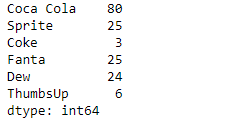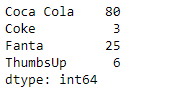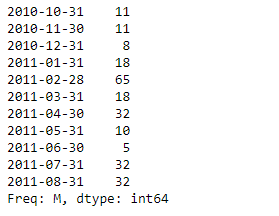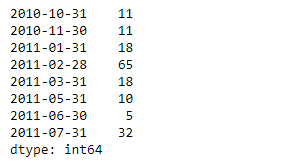# Python | Pandas Series.drop()

Pandas series is a One-dimensional ndarray with axis labels. The labels need not be unique but must be a hashable type. The object supports both integer- and label-based indexing and provides a host of methods for performing operations involving the index.

Pandas` Series.drop()` function return Series with specified index labels removed. It remove elements of a Series based on specifying the index labels.

Syntax: Series.drop(labels=None, axis=0, index=None, columns=None, level=None, inplace=False, errors=’raise’)

Parameter :
labels : Index labels to drop.
axis : Redundant for application on Series.
index, columns : Redundant for application on Series, but index can be used instead of labels.
level : For MultiIndex, level for which the labels will be removed.
inplace : If True, do operation inplace and return None.
errors : If ‘ignore’, suppress error and only existing labels are dropped.

Returns : dropped : pandas.Series

Example #1: Use `Series.drop()` function to drop the values corresponding to the passed index labels in the given series object.

 `# importing pandas as pd ` `import` `pandas as pd ` ` `  `# Creating the Series ` `sr ``=` `pd.Series([``80``, ``25``, ``3``, ``25``, ``24``, ``6``]) ` ` `  `# Create the Index ` `index_ ``=` `[``'Coca Cola'``, ``'Sprite'``, ``'Coke'``, ``'Fanta'``, ``'Dew'``, ``'ThumbsUp'``] ` ` `  `# set the index ` `sr.index ``=` `index_ ` ` `  `# Print the series ` `print``(sr) `

Output :Now we will use `Series.drop()` function to drop the values corresponding to the passed index labels in the given series object.

 `# drop the passed labels ` `result ``=` `sr.drop(labels ``=` `[``'Sprite'``, ``'Dew'``])  ` ` `  `# Print the result ` `print``(result) `

Output :As we can see in the output, the `Series.drop()` function has successfully dropped the entries corresponding to the passed index labels.

Example #2 : Use `Series.drop()` function to drop the values corresponding to the passed index labels in the given series object.

 `# importing pandas as pd ` `import` `pandas as pd ` ` `  `# Creating the Series ` `sr ``=` `pd.Series([``11``, ``11``, ``8``, ``18``, ``65``, ``18``, ``32``, ``10``, ``5``, ``32``, ``32``]) ` ` `  `# Create the Index ` `index_ ``=` `pd.date_range(``'2010-10-09'``, periods ``=` `11``, freq ``=``'M'``) ` ` `  `# set the index ` `sr.index ``=` `index_ ` ` `  `# Print the series ` `print``(sr) `

Output :Now we will use `Series.drop()` function to drop the values corresponding to the passed index labels in the given series object.

 `# drop the passed labels ` `result ``=` `sr.drop(labels ``=` `[pd.Timestamp(``'2010-12-31'``), ` `                           ``pd.Timestamp(``'2011-04-30'``), pd.Timestamp(``'2011-08-31'``)]) ` ` `  `# Print the result ` `print``(result) `

Output :As we can see in the output, the `Series.drop()` function has successfully dropped the entries corresponding to the passed index labels.

My Personal Notes arrow_drop_upCheck out this Author's contributed articles.

If you like GeeksforGeeks and would like to contribute, you can also write an article using contribute.geeksforgeeks.org or mail your article to contribute@geeksforgeeks.org. See your article appearing on the GeeksforGeeks main page and help other Geeks.

Please Improve this article if you find anything incorrect by clicking on the "Improve Article" button below.

Article Tags :

Be the First to upvote.

Please write to us at contribute@geeksforgeeks.org to report any issue with the above content.